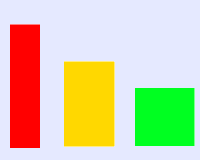# Working Systematically

We have chosen a selection of our favourite NRICH resources that challenge you to work systematically.Here are a few questions you might need to ask along the way:

Which piece of information is the most useful to start with? What next?
How will I record my findings?
Will I be confident that I have found all the results by the end?

The problems can be tackled in any order.### Two and Two

##### Age 11 to 16Challenge Level

How many solutions can you find to this sum? Each of the different letters stands for a different number.### 1 Step 2 Step

##### Age 11 to 14Challenge Level

Liam's house has a staircase with 12 steps. He can go down the steps one at a time or two at time. In how many different ways can Liam go down the 12 steps?### ACE, TWO, THREE...

##### Age 11 to 14Challenge Level

Can you picture how to order the cards to reproduce Charlie's card trick for yourself?### Isosceles Triangles

##### Age 11 to 14Challenge Level

Draw some isosceles triangles with an area of $9$cm$^2$ and a vertex at (20,20). If all the vertices must have whole number coordinates, how many is it possible to draw?### M, M and M

##### Age 11 to 14Challenge Level

If you are given the mean, median and mode of five positive whole numbers, can you find the numbers?### Can They Be Equal?

##### Age 11 to 14Challenge Level

Can you find rectangles where the value of the area is the same as the value of the perimeter?### Sticky Numbers

##### Age 11 to 14Challenge Level

Can you arrange the numbers 1 to 17 in a row so that each adjacent pair adds up to a square number?### Shifting Times Tables

##### Age 11 to 14Challenge Level

Can you find a way to identify times tables after they have been shifted up or down?### Charlie's Delightful Machine

##### Age 11 to 16Challenge Level

Here is a machine with four coloured lights. Can you develop a strategy to work out the rules controlling each light?### American Billions

##### Age 11 to 14Challenge Level

Play the divisibility game to create numbers in which the first two digits make a number divisible by 2, the first three digits make a number divisible by 3...